Try NerdPal! Our new app on iOS and Android

# Solve the equation $\frac{x+1}{2}-\frac{2-x}{3}=0$

## Related Videos

Go!
Go!
1
2
3
4
5
6
7
8
9
0
a
b
c
d
f
g
m
n
u
v
w
x
y
z
.
(◻)
+
-
×
◻/◻
/
÷
2

e
π
ln
log
log
lim
d/dx
Dx
|◻|
θ
=
>
<
>=
<=
sin
cos
tan
cot
sec
csc

asin
acos
atan
acot
asec
acsc

sinh
cosh
tanh
coth
sech
csch

asinh
acosh
atanh
acoth
asech
acsch

### Videos### Solving for F in terms of C | Linear equations | Algebra I | Khan Academy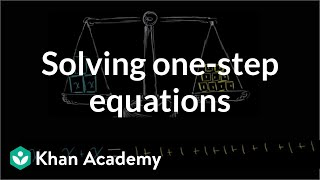### Intuition for solving one-step equations | Linear equations | Algebra I | Khan Academy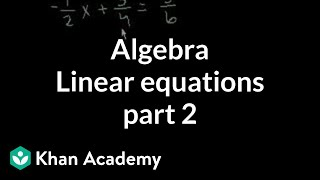### Algebra: Linear equations 2 | Linear equations | Algebra I | Khan Academy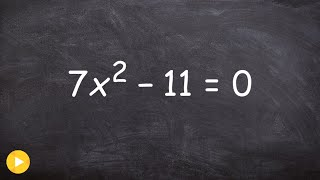### Pre-Calculus - Using square root method to solve a quadratic equation with one variable, 7x^2 -11=0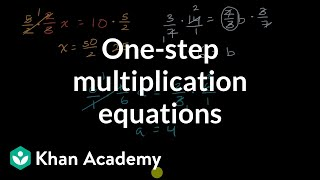### How to solve equations of the form x/a = b | Linear equations | Algebra I | Khan Academy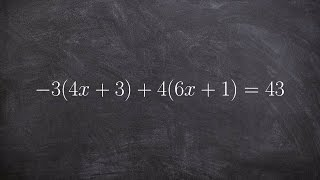SnapXam A2

Go!
1
2
3
4
5
6
7
8
9
0
a
b
c
d
f
g
m
n
u
v
w
x
y
z
.
(◻)
+
-
×
◻/◻
/
÷
2

e
π
ln
log
log
lim
d/dx
Dx
|◻|
θ
=
>
<
>=
<=
sin
cos
tan
cot
sec
csc

asin
acos
atan
acot
asec
acsc

sinh
cosh
tanh
coth
sech
csch

asinh
acosh
atanh
acoth
asech
acsch

### Tips on how to improve your answer:

$\frac{x+1}{2}-\frac{2-x}{3}=0$

### Main topic:

One-variable linear equations

~ 0.07 s